PROC PLS Statement
PROC PLS <options> ;

You use the PROC PLS statement to invoke the PLS procedure and, optionally, to indicate the analysis data and method. The following options are available.

CENSCALE

lists the centering and scaling information for each response and predictor.

CV=ONE
CV=SPLIT <(n)>
CV=BLOCK <(n)>
CV=RANDOM <(cv-random-opts)>
CV=TESTSET(SAS-data-set)

specifies the cross validation method to be used. By default, no cross validation is performed. The method CV=ONE requests one-at-a-time cross validation, CV=SPLIT requests that everyth observation be excluded, CV=BLOCK requests thatblocks of consecutive observations be excluded, CV=RANDOM requests that observations be excluded at random, and CV=TESTSET(SAS-data-set) specifies a test set of observations to be used for validation (formally, this is called "test set validation" rather than "cross validation"). You can, optionally, specifyfor CV=SPLIT and CV=BLOCK; the default is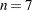. You can also specify the following optional cv-random-options in parentheses after the CV=RANDOM option:

NITER=n

specifies the number of random subsets to exclude. The default value is 10.

NTEST=n

specifies the number of observations in each random subset chosen for exclusion. The default value is one-tenth of the total number of observations.

SEED=n

specifies an integer used to start the pseudo-random number generator for selecting the random test set. If you do not specify a seed, or specify a value less than or equal to zero, the seed is by default generated from reading the time of day from the computer’s clock.

CVTEST <(cvtest-options)>

specifies that van der Voet’s (1994) randomization-based model comparison test be performed to test models with different numbers of extracted factors against the model that minimizes the predicted residual sum of squares; see the section Cross Validation for more information. You can also specify the following cv-test-options in parentheses after the CVTEST option:

PVAL=n

specifies the cutoff probability for declaring an insignificant difference. The default value is 0.10.

STAT=test-statistic

specifies the test statistic for the model comparison. You can specify either T2, for Hotelling’s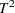statistic, or PRESS, for the predicted residual sum of squares. The default value is T2.

NSAMP=n

specifies the number of randomizations to perform. The default value is 1000.

SEED=n

specifies the seed value for randomization generation (the clock time is used by default).

DATA=SAS-data-set

names the SAS data set to be used by PROC PLS. The default is the most recently created data set.

DETAILS

lists the details of the fitted model for each successive factor. The details listed are different for different extraction methods; see the section Displayed Output for more information.

METHOD=PLS <( PLS-options )>
METHOD=SIMPLS
METHOD=PCR
METHOD=RRR

specifies the general factor extraction method to be used. The value PLS requests partial least squares, SIMPLS requests the SIMPLS method of de Jong (1993), PCR requests principal components regression, and RRR requests reduced rank regression. The default is METHOD=PLS. You can also specify the following optional PLS-options in parentheses after METHOD=PLS:

ALGORITHM=NIPALS | SVD | EIG | RLGW

names the specific algorithm used to compute extracted PLS factors. NIPALS requests the usual iterative NIPALS algorithm, SVD bases the extraction on the singular value decomposition of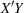, EIG bases the extraction on the eigenvalue decomposition of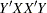, and RLGW is an iterative approach that is efficient when there are many predictors. ALGORITHM=SVD is the most accurate but least efficient approach; the default is ALGORITHM=NIPALS.

MAXITER=n

specifies the maximum number of iterations for the NIPALS and RLGW algorithms. The default value is 200.

EPSILON=n

specifies the convergence criterion for the NIPALS and RLGW algorithms. The default value is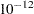.

MISSING=NONE
MISSING=AVG
MISSING=EM <( EM-options )>

specifies how observations with missing values are to be handled in computing the fit. The default is MISSING=NONE, for which observations with any missing variables (dependent or independent) are excluded from the analysis. MISSING=AVG specifies that the fit be computed by filling in missing values with the average of the nonmissing values for the corresponding variable. If you specify MISSING=EM, then the procedure first computes the model with MISSING=AVG and then fills in missing values by their predicted values based on that model and computes the model again. For both methods of imputation, the imputed values contribute to the centering and scaling values, and the difference between the imputed values and their final predictions contributes to the percentage of variation explained. You can also specify the following optional EM-options in parentheses after MISSING=EM:

MAXITER=n

specifies the maximum number of iterations for the imputation/fit loop. The default value is 1. If you specify a large value of MAXITER=, then the loop will iterate until it converges (as controlled by the EPSILON= option).

EPSILON=n

specifies the convergence criterion for the imputation/fit loop. The default value is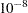. This option is effective only if you specify a large value for the MAXITER= option.

NFAC=n

specifies the number of factors to extract. The default is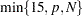, whereis the number of predictors (the number of dependent variables for METHOD=RRR) and
is the number of runs (observations). This is probably more than you need for most applications. Extracting too many factors can lead to an overfit model, one that matches the training data too well, sacrificing predictive ability. Thus, if you use the default NFAC= specification, you should also either use the CV= option to select the appropriate number of factors for the final model or consider the analysis to be preliminary and examine the results to determine the appropriate number of factors for a subsequent analysis.

NOCENTER

suppresses centering of the responses and predictors before fitting. This is useful if the analysis variables are already centered and scaled. See the section Centering and Scaling for more information.

NOCVSTDIZE

suppresses re-centering and rescaling of the responses and predictors before each model is fit in the cross validation. See the section Centering and Scaling for more information.

NOPRINT

suppresses the normal display of results. This is useful when you want only the output statistics saved in a data set. Note that this option temporarily disables the Output Delivery System (ODS); see Chapter 20, Using the Output Delivery System for more information.

NOSCALE

suppresses scaling of the responses and predictors before fitting. This is useful if the analysis variables are already centered and scaled. See the section Centering and Scaling for more information.

PLOTS <(global-plot-options)> <= plot-request <(options)>>
PLOTS <(global-plot-options)> <= (plot-request <(options)> <... plot-request <(options)>>)>

controls the plots produced through ODS Graphics. When you specify only one plot request, you can omit the parentheses from around the plot request. For example:

```plots=none
plots=cvplot
plots=(diagnostics cvplot)
plots(unpack)=diagnostics
```

ODS Graphics must be enabled before requesting plots. For example:

```ods graphics on;
proc pls data=pentaTrain;
model log_RAI = S1-S5 L1-L5 P1-P5;
run;
ods graphics off;
```

For more information about enabling and disabling ODS Graphics, see the section Enabling and Disabling ODS Graphics in Chapter 21, Statistical Graphics Using ODS.

If ODS Graphics is enabled but you do not specify the PLOTS= option, then PROC PLS produces by default a plot of the R-square analysis and a correlation loading plot summarizing the first two factors.

The global plot options include the following:

FLIP

interchanges the X-axis and Y-axis dimensions for the score, weight, and loading plots.

ONLY

suppresses the default plots. Only plots specifically requested are displayed.

UNPACKPANEL
UNPACK

suppresses paneling. By default, multiple plots can appear in some output panels. Specify UNPACKPANEL to get each plot in a separate panel. You can specify PLOTS(UNPACKPANEL) to unpack only the default plots. You can also specify UNPACKPANEL as a suboption for certain specific plots, as discussed in the following.

The plot requests include the following:

ALL

produces all appropriate plots. You can specify other options with ALL—for example, to request all plots and unpack only the residuals, specify PLOTS=(ALL RESIDUALS(UNPACK)).

CORRLOAD <(TRACE = ON | OFF)>

produces a correlation loading plot (default). The TRACE= option controls how points corresponding to the X-loadings in the correlation loadings plot are depicted. By default, these points are depicted by the name of the corresponding model effect if there are 20 or fewer of them; otherwise, they are depicted by a connected "trace" through the points. You can use this option to change this behavior.

CVPLOT

produces a cross validation and R-square analysis. This plot requires the CV= option to be specified, and is displayed by default in this case.

DIAGNOSTICS <(UNPACK)>

produces a summary panel of the fit for each dependent variable. The summary by default consists of a panel for each dependent variable, with plots depicting the distribution of residuals and predicted values. You can use the UNPACK suboption to specify that the subplots be produced separately.

DMOD

produces the DMODX, DMODY, and DMODXY plots.

DMODX

produces a plot of the distance of each observation to the X model.

DMODXY

produces plots of the distance of each observation to the X and Y models.

DMODY

produces a plot of the distance of each observation to the Y model.

FIT

produces both the fit diagnostic panel and the ParmProfiles plot.

NONE

suppresses the display of graphics.

PARMPROFILES

produces profiles of the regression coefficients.

SCORES <(UNPACK | FLIP)>

produces the XScores, YScores, XYScores, and DModXY plots. You can use the UNPACK suboption to specify that the subplots for scores be produced separately, and the FLIP option to interchange their default X-axis and Y-axis dimensions.

RESIDUALS <(UNPACK)>

plots the residuals for each dependent variable against each independent variable. Residual plots are by default composed of multiple plots combined into a single panel. You can use the UNPACK suboption to specify that the subplots be produced separately.

VIP

produces profiles of variable importance factors.

WEIGHTS <(UNPACK | FLIP)>

produces all X and Y loading and weight plots, as well as the VIP plot. You can use the UNPACK suboption to specify that the subplots for weights and loadings be produced separately, and the FLIP option to interchange their default X-axis and Y-axis dimensions.

produces a scatter plot matrix of X-loadings against each other. Loading scatter plot matrices are by default composed of multiple plots combined into a single panel. You can use the UNPACK suboption to specify that the subplots be produced separately, and the FLIP option to interchange the default X-axis and Y-axis dimensions.

XSCORES <(UNPACK | FLIP)>

produces a scatter plot matrix of X-scores against each other. Score scatter plot matrices are by default composed of multiple plots combined into a single panel. You can use the UNPACK suboption to specify that the subplots be produced separately, and the FLIP option to interchange the default X-axis and Y-axis dimensions.

XWEIGHTPLOT <(UNPACK | FLIP)>

produces a scatter plot matrix of X-weights against each other. Weight scatter plot matrices are by default composed of multiple plots combined into a single panel. You can use the UNPACK suboption to specify that the subplots be produced separately, and the FLIP option to interchange the default X-axis and Y-axis dimensions.

XWEIGHTPROFILES

produces profiles of the X-weights.

XYSCORES <(UNPACK)>

produces a scatter plot matrix of X-scores against Y-scores. Score scatter plot matrices are by default composed of multiple plots combined into a single panel. You can use the UNPACK suboption to specify that the subplots be produced separately.

YSCORES <(UNPACK | FLIP)>

produces a scatter plot matrix of Y-scores against each other. Score scatter plot matrices are by default composed of multiple plots combined into a single panel. You can use the UNPACK suboption to specify that the subplots be produced separately, and the FLIP option to interchange the default X-axis and Y-axis dimensions.

YWEIGHTPLOT <(UNPACK | FLIP)>

produces a scatter plot matrix of Y-weights against each other. Weight scatter plot matrices are by default composed of multiple plots combined into a single panel. You can use the UNPACK suboption to specify that the subplots be produced separately, and the FLIP option to interchange the default X-axis and Y-axis dimensions.

VARSCALE

specifies that continuous model variables be centered and scaled prior to centering and scaling the model effects in which they are involved. The rescaling specified by the VARSCALE option is sometimes more appropriate if the model involves crossproducts between model variables; however, the VARSCALE option still might not produce the model you expect. See the section Centering and Scaling for more information.